# Chemical Kinetics Rate and Order Notes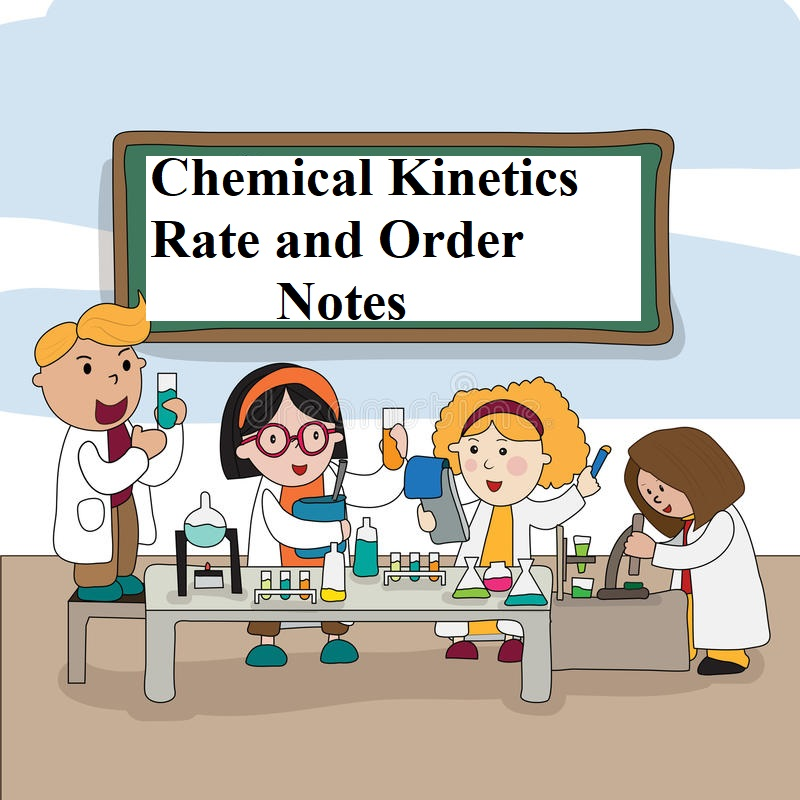Chemical Kinetics Rate and Order Notes

Chemical Kinetics Rate and Order Notes:

Chemical Kinetics: The branch of Chemistry that deals with the rate of chemical reaction, factors affecting the rate, and the mechanism of a reaction.
Types of Reactions: On the basis of rates
(i) Very fast reactions – e.g. precipitation of AgCl
(ii) Very slow reactions – e.g. rusting of iron
(iii) Reactions taking place at moderate speeds – e.g. hydrolysis of starch

Rate of Chemical Reaction: It is the change in concentration of reactant or product with respect to time.
OR
It is the rate of decrease in the concentration of any one of the reactants or the rate of increase in the concentration of any one of the products.
For a chemical reaction, if ∆x is the change in concentration of a reactant or a product in a time ∆t, the rate of reaction (r) = ∆x/∆t.  For a hypothetical reaction R → P, the rate of reaction can be expressed by a decrease in the concentration of reactants or by an increase in the concentration of products.
Rate of reaction = Rate of disappearance of R

r = Decrease in concentration of R =   – ∆[R]
Time is taken                          ∆t
ve sign before ∆[R] indicate the decrease in the concentration of Reactant

OR

Rate of reaction = Rate of appearance (formation) of P
r = Increase in concentration of P = ∆[P]
Time is taken                       ∆t

Concentration is expressed in moles/L and time is in second, the unit of rate of reaction is mol/L/s.

Types of Rate of Reaction:
The average rate of reaction: The rate of reaction measured over the long time interval is called the average rate of reaction.
Avg rate ∆x = – ∆[R]  =   ∆[P]
∆t        ∆t           ∆t

Chemical Kinetics Rate and Order Notes

Instantaneous rate of reaction: The rate of reaction measured at a particular time is called the instantaneous rate of reaction.
Instantaneous rate (rinst) dx = – d[R]  =   d[P]      (∆t → 0)
dt         dt           dt

The average rate becomes Instantaneous rate when ∆t approaches zero.

Overall Rate of a General Reaction:
When there are several reactants and products the individual rates of the various components may differ as they would depend on the stoichiometric coefficients.
For a reaction,
A + 2B → 3C + 4D
Rate of disappearance of B = 2 × Rate of disappearance of A (2:1)
Rate of formation of C = 3 × Rate of disappearance of A (3:1)
Rate of formation of D = 4 × Rate of disappearance of A (4:1)

Factors Affecting the Rate of Reaction:
(i) Concentration of reactant
(ii) Surface area
(iii) Temperature
(iv) Nature of reactant
(v) Presence of catalyst.

Dependence rate on Concentration:
Rate Law: The rate of reaction is directly proportional to the product of the concentration of reactant and each concentration is raised to some power which may or may not be equal to stereochemistry experimentally.
For a general reaction, aA + bB → cC + dD,
Rate α [A]x[B]y
Rate = k[A]x[B]y (Where x and y may or may not equal to a & b).
k is proportionality constant and is called Rate Constant

Rate Constant (k): Rate constant is a rate of reaction when the molar concentration of reactant is unity.

Molecularity of a Reaction: The no. of molecules of the reactants involved in the reaction is known as Molecularity. It cannot be zero or fractional. It can have values 1,2,3 etc. it is applicable only to elementary reactions.
If the molecularity of a reaction is 1, it is called Unimolecular Reaction. e.g. Decomposition of ammonium nitrite NH4NO2 → N2 + 2 H2O.
If the molecularity of a reaction is 2, it is called a Bimolecular Reaction. e.g. Decomposition of Hydrogen Iodide   2 HI → H2 + I2

Order of Reaction: Order is the sum of the powers of the concentration terms of the reactants in the rate law. It is an experimental quantity. It can have the values 0,1,2,3… or a fraction. It is applicable to both elementary and complex reactions.
For a general reaction, aA + bB → cC + dD
r = k[A]x[B]y, Order of the reaction = x + y
If the order of a reaction is zero, it is called Zero Order Reaction, if it is one, it is called First Order Reaction, if it is two, it is called Second Order Reaction, and so on.

 S.No. Order Molecularity 1. It is the sum of the powers of the concentration terms in the rate law expression. It is the total number of reactant species that collide simultaneously in a chemical reaction. 2. It is an experimental quantity. It is a theoretical quantity. 3. It can be zero or fractional. It cannot be zero or fractional. 4. It is applicable to both elementary and complex reactions. It is applicable only to elementary reactions.

Unit of Rate Constant:
Different ordered reactions have different units for k.
For an nth order reaction
For the reaction, nA → Products
rate =  k[A]n
Therefore, k =     rate          ………………….(1)
[Concentration]n
From relation (1) we can find the order for various reactions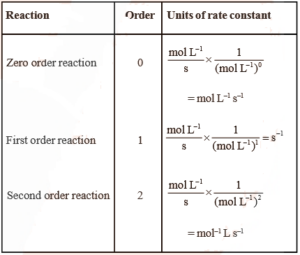Integrated Rate Equations: These are equations relating to the rate of a reaction and the concentration of reactants. Different ordered reactions have different integrated rate law equations.

For Zero Order Reaction: Zero-order reactions are reactions in which the rate of reaction is proportional to the zero power of the concentration of reactants.
Consider a zero-order reaction     R → P
The rate expression for the above reaction is
r = – d[R] ………………. (1)
dt
The rate law for the above reaction is
r= k[R]0 = k ………………… (2)
From equations (1) & (2), we can write
k    =   – d[R]                                                                                                                                               dt
The above equation is known as the differential rate equation for the zero-order reaction.
d[R] = – kdt
On integrating the above equation, we get
[R] = – kt + C      …………………. (3)
Where C is the constant of integration. To calculate the value of C, consider the initial conditions. i.e., when t=0, [R] = [R]0
Substitute these values in equation (3)
[R]0 = – k × 0 + C
C = – [R]0
Substituting C in equation (3), we get
[R] = – kt  –  [R]0  …………. (4)
[R]0 – [R] = kt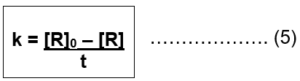This equation is of the form of a straight line y = mx + c. So if we plot [R] against t, we get a straight line with slope = – k and intercept equal to [R]0.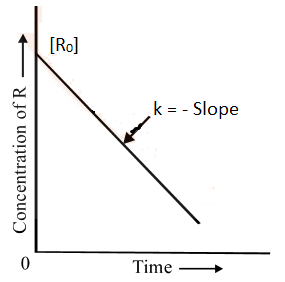The decomposition of gaseous ammonia on a hot platinum surface at high pressure.
N2 + H2 → 2 NH3 At high pressure, molecules. So, a further change in reaction conditions does not change the rate of the reaction. So, it becomes a zero-order reaction.
Another e.g. is the thermal decomposition of HI on a gold surface

For First Order Reaction: First-order reactions are reactions in which the rate of reaction is proportional to the first power of the concentration of reactants.
Consider a first order reaction     R → P
The rate expression for the above reaction is
r = – d[R] ………………. (1)
dt
Rate law for the above reaction is
r= k[R]1 = k ………………… (2)
From equations (1) & (2), we can write
k [R] =   – d[R]
dt
d[R]   = – k dt
[R]
On integrating the above equation, we get
In [R] = – kt + C …….. (3)
Where C is the constant of integration. To calculate the value of C, consider the initial conditions. i.e., when t=0, [R] = [R]0
Substitute these values in equation (3)
In [R0] = – k × 0 + C
C = ln [R0]
Substituting C in equation (3), we get
ln[R] = – kt + ln[R0] ………… (4)
Rearranging above equation we get
kt = ln[R0] – ln[R]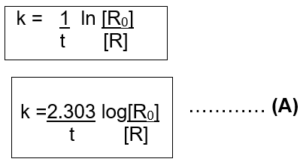At time t1 from equation (4)
In [R1] = – kt1 + ln [R2]
At time t2
ln [R1] = – kt2 + ln [R2]
where [R1] and [R2] are the concentrations of the reactants at time t1 and t2 respectively.
Subtracting
ln [R1] – ln [R2] = – kt1 – (–kt2)
ln [R1] = k (t2 – t1)
[R2]
Comparing equation (2) with y = mx + c, if we plot In [R] against t, we get a straight line with slope = – k and intercept equal to ln [R0].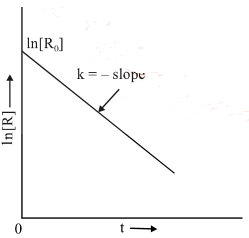The first-order rate equation (A) can also be written in the form
Log [R0] =     kt
[R]      2.303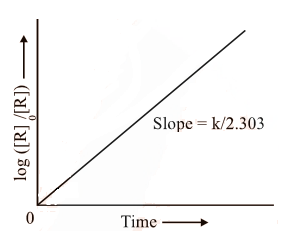For Example: Hydrogenation of ethene:
C2H4(g) + H2(g) → C2H6(g);     r = k[C2H4]

PSEUDO FIRST ORDER REACTION
https://www.sciencemotive.com/class-12-chemistry-2/pseudo-first-order-reaction/

Chemical Kinetics Rate and Order Notes